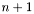# String conversions and I/O

## Functions

int fmpz_poly_q_from_string (fmpz_poly_q_ptr rop, const char *s)
char * fmpz_poly_q_to_string (const fmpz_poly_q_ptr op)
char * fmpz_poly_q_to_string_pretty (const fmpz_poly_q_ptr op, const char *x)
void fmpz_poly_q_print (const fmpz_poly_q_ptr op)
void fmpz_poly_q_print_pretty (const fmpz_poly_q_ptr op, const char *x)

## Detailed Description

The following three methods enable users to construct elements of type `fmpz_poly_q_ptr` from strings or to obtain string representations of such elements.

The format used is based on the FLINT format for integer polynomials of type `fmpz_poly_t`, which we recall first:

A non-zero polynomialof lengthis represented by the string `n+1 a_0 a_1 ... a_n`, where there are two space characters following the length and single space characters separating the individual coefficients. There is no leading or trailing white-space. In contrast, the zero polynomial is represented by `0`.

We adapt this notation for rational functions as follows. We denote the zero function by `0`. Given a non-zero function with numerator and denominator string representations `num` and `den`, respectively, we use the string `num/den` to represent the rational function, unless the denominator is equal to one, in which case we simply use `num`.

There is also a `_pretty` variant available, which bases the string parts for the numerator and denominator on the output of the function `fmpz_poly_to_string_pretty` and introduces parentheses where necessary.

Note that currently these functions are not optimized for performance and are intended to be used only for debugging purposes or one-off input and output, rather than as a low-level parser.

## Function Documentation

 int fmpz_poly_q_from_string ( fmpz_poly_q_ptr rop, const char * s )

Sets the rational function `rop` to the value specified by the null-terminated string `s`.

This method has now already been somewhat improved and is not very tolerant in the handling of malformed input. It expects either legitimate input for an `fmpz_poly_t` element, or two such inputs separated by a `/` only, in which case it is also assumed that the second polynomial is non-zero.

The rational function is brought into canonical form by calling fmpz_poly_q_canonicalize() in this function.

Returns `1` if the string represents a valid rational function and `0` otherwise.

 void fmpz_poly_q_print ( const fmpz_poly_q_ptr op )

Prints the standard non-pretty representation of `op` to `stdout`.

 void fmpz_poly_q_print_pretty ( const fmpz_poly_q_ptr op, const char * x )

Prints the standard pretty representation of `op` to `stdout`.

 char* fmpz_poly_q_to_string ( const fmpz_poly_q_ptr op )

Returns the string representation of the rational function `op`.

 char* fmpz_poly_q_to_string_pretty ( const fmpz_poly_q_ptr op, const char * x )

Returns the pretty string representation of `op`.

Returns the pretty string representation of the rational function `op`, using the string `x` as the variable name.

Generated on Wed Dec 8 17:00:29 2010 for FMPZ_POLY_Q by1.6.3